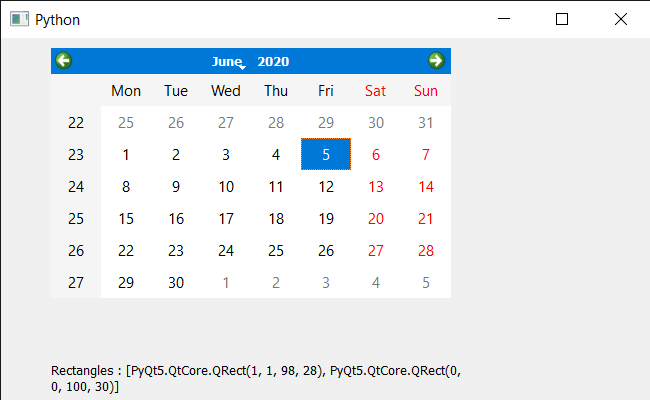# PyQt5 QCalendarWidget – Accessing Rectangle of each children if possible

• Last Updated : 20 Sep, 2021

In this article we will see how we get the rectangle of all the children of QCalendarWidget. Calendar is not alone a single widget it is a mixture of lots of smaller widget which we refer as calendar’s child. There are many child such as table view, item delegate etc, we use children method to get all the children of it. Rectangle is the bounding rectangle of each children.
Note : All children don’t have rectangle as they can be a layout.

In order to do this we have to do the following :
1. Create a calendar widget
2. Get all the children of the calendar
3. Traverse the list of children
4. Try to get the rectangle in the try, except block and save result in another list
5. Show the list using label

Below is the implementation

## Python3

 `# importing libraries``from` `PyQt5.QtWidgets ``import` `*``from` `PyQt5 ``import` `QtCore, QtGui``from` `PyQt5.QtGui ``import` `*``from` `PyQt5.QtCore ``import` `*``import` `sys`  `class` `Window(QMainWindow):` `    ``def` `__init__(``self``):``        ``super``().__init__()` `        ``# setting title``        ``self``.setWindowTitle(``"Python "``)` `        ``# setting geometry``        ``self``.setGeometry(``100``, ``100``, ``650``, ``400``)` `        ``# calling method``        ``self``.UiComponents()` `        ``# showing all the widgets``        ``self``.show()` `    ``# method for components``    ``def` `UiComponents(``self``):` `        ``# creating a QCalendarWidget object``        ``self``.calendar ``=` `QCalendarWidget(``self``)` `        ``# setting geometry to the calendar``        ``self``.calendar.setGeometry(``50``, ``10``, ``400``, ``250``)` `        ``# creating a label``        ``label ``=` `QLabel(``self``)` `        ``# setting geometry``        ``label.setGeometry(``50``, ``280``, ``420``, ``120``)` `        ``# making it multi line``        ``label.setWordWrap(``True``)` `        ``# getting children``        ``children ``=` `self``.calendar.children()`  `        ``value ``=` `[]``        ``for` `i ``in` `children:``            ``try``:``                ` `                ``try``:``                    ``# getting children rectangle``                    ``rect ``=` `i.childrenRect()``                ``except``:``                    ``# getting rectangle``                    ``rect ``=` `i.rect()``                    ` `                ``# adding it to list``                ``value.append(rect)` `            ``except``:``                ``pass` `        ``# setting text to the label``        ``label.setText(``"Rectangles : "` `+` `str``(value))`   `# create pyqt5 app``App ``=` `QApplication(sys.argv)` `# create the instance of our Window``window ``=` `Window()`  `# start the app``sys.exit(App.``exec``())`

Output :My Personal Notes arrow_drop_up